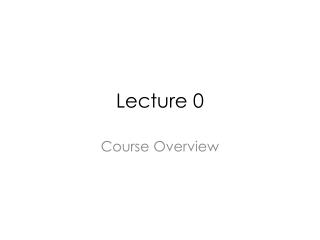Download PresentationLecture 0

# Lecture 0

Download Presentation## Lecture 0

- - - - - - - - - - - - - - - - - - - - - - - - - - - E N D - - - - - - - - - - - - - - - - - - - - - - - - - - -
##### Presentation Transcript

1. Lecture 0 Course Overview

2. ES 345/485 Engineering Probability • Course description: Probability and its axioms, conditional probability, sequential experiments, independence, counting, discrete, continuous distributions, functions of random variables, expectations, multiple random variables and joint distributions, central limit theorem, weak law of large numbers, estimation of random variables. • When: • TTH 4:00-5:15 (Lectures) • Classroom: • Salazar Hall 2001

3. Course Webpage

4. Instructor Availability • Instructor: Dr. Jack Ou • Office: Salazar 2010B • Office hours: • MW 10:30-11:00, TH 3:00 to 4:00 • Email: jack.ou@sonoma.edu • I like to communicate over email. • I do not check my voice mail. • Do not overlook the opportunity to meet with the instructor.

5. Prerequisite: Math 211 • Chapter 1: factorial • Chapter 2: natural log, exponential function, finite geometric series, infinite geometric series, • Chapter 3: concepts of limits, fundamental theorem of calculus, integration by parts • Chapter 4: double integration, double summation. • Chapter 6: moment generating function (similar to Laplace transform)

6. Khan Academy • Useful reference: • https://www.khanacademy.org/math/probability • https://www.khanacademy.org/math/differential-calculus • https://www.khanacademy.org/math/integral-calculus

7. Textbook • (Required) Roy Yates and David Goodman, “Probability and Stochastic Processes: A Friendly Introduction for Electrical and Computer Engineers,” Second Edition, 2005. ISBN 978-0-471-27214-4. • (Required) W. Michael Kelly and Robert A. Donnelly, “The Humongous Book of Statistics Problems,” 2009. ISBN 978-1-59257-865-8. • (Optional) Larry Gonick and Woollcott Smith, “The Cartoon Guide to Statistics.” • (Optional) Jim Albert, “Teaching Statistics Using Baseball.”

8. Chapter 1 • Develop basic probability vocabulary. • Example:

9. Chapter 2: Discrete Random Variable

10. Chapter 3: Continuous Random Variable

11. Concepts that permeate throughout the book

12. Chapter 4: Functions of Two Random Variable

13. Chapter 6: Central Limit Theorem • Typical Problem: A modem transmits one million bits. What is the probability that there are at least 499,000 ones, but no more than 501,000 ones?

14. Chapter 7 : Confidence Intervals • Typical problem: Based on a sample of 1103 voters, the percentage of people supporting Jerry Brown is with an accuracy of plus or minus 3 percentage points.

15. Hypothesis Testing

16. Course Policies • Regarding classroom conduct • Regarding homework, exams and Piazza

17. Grading • Three exams • (total: 60 %. Exam #1=10 %, exam #2=20%, and final=30 %) • Class participation • (total: 10 %. Attendance=5%, participate in active learning exercises both in class and out of class=5%) • Project • 10 % • Homework assignment: • 20 % Reminder: ES230 is a 3 unit course requiring an average of 9-12 hours of study per week!

18. Classroom Participation • Examples of exercises • You are given a blank sheet of paper, and asked to respond a brief question. You will not be graded. • You maybe asked to present the solution you have worked on in class. • You maybe asked to summarized other students’ response • You maybe asked to complete anonymous survey about the course

19. Homework • 20 % of the final grade • Problems in each assignment are graded selectively. • Effort based. • Each assignment can take on an average four hours to complete • Please start your assignment early. Do not wait till the last minute • Post your questions on Piazza

20. Homework Tips • Try to attack the problems from different angles • Ask the what if questions • Summarize the question in your own words. • “I am stuck because …..” • “This problem frustrates me because….” • “Here is what I do not understand…..” • “Here is what I know about this problem….” • Be self-critical. Be suspicious of assumptions that you considered to be true. • Be disciplined. If you are truly stuck, move on and come back to work on the question.

21. Tests • Review sheet

22. Helpful practices for better grades • Before class • Download the lecture notes from the website and review them • Make a list of questions that you want to ask • Make use of instructor’s office hour • In class • Stay awake and listen actively • Participate in exercises • Take notes. Make a mental list on what is confusing to you. • After class • Set aside a time to study. • Review the notes . Use the textbook as a reference. • Do assignments/homework before the deadline • Post questions on Piazza

23. Dates • 9/3 last day to drop a course with adjusted fees (done on-line) • 9/4-15: drop with a “W” (done on-line) • 9/16-11/13: petition to withdraw from a class with \$20 administrative fee (dropping classes permitted because of serious and compelling reasons)